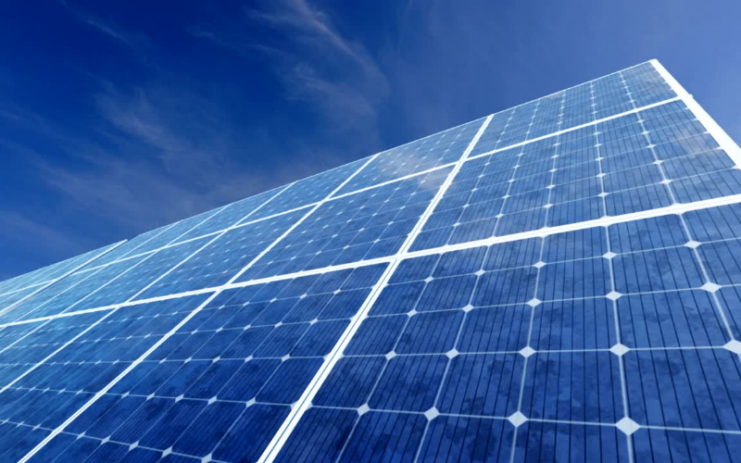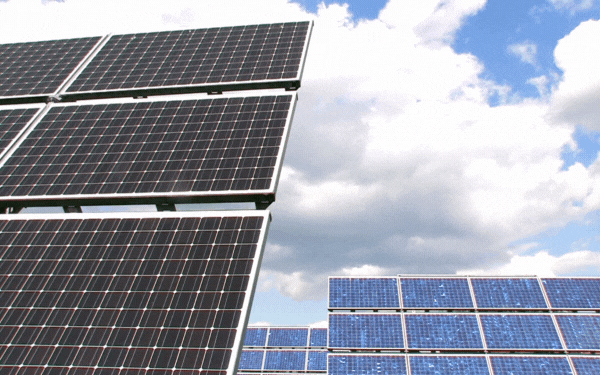# How Many Solar Panels Are Needed to Power the US? – Number of Panels to Cover Land

The question ‘how many solar panels power the US?’ is a good one since it will likely become an essential issue in the coming years. By looking at this question, we need to figure out how much power the United States consumes annually.

Then, we need to look at how much electricity the average acre of a solar panel is. We also need to take into account things like land space and the number of panels required to cover that land. Let us get started.

## How Many Solar Panels Are Needed to Power the US?### How Many Acres Does It Take to Produce a GWh?

It takes roughly three acres of solar panels working to produce a gigawatt every hour for a year.

### How Much Energy Does the US Produce Annually?

The United States produces roughly four petawatts of energy annually. One petawatt is the equivalent of one billion million watts. That is a lot of watts!